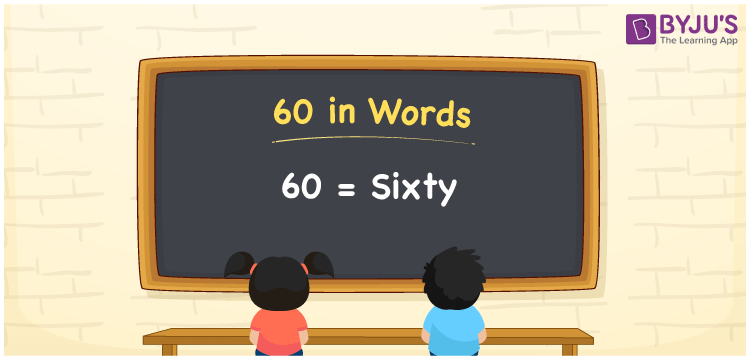# 60 in Words

60 in words is written as “Sixty”. In Mathematics, 60 is a natural number that is used to represent a value. To express the number we use English alphabets and write them in words. Hence, 60 is given by Sixty in English. For example, Joy brought a pen worth Rs.60 or Rupees Sixty. Learn more about Numbers In Words at BYJU’S.

 60 in Words Sixty Sixty in Numbers 60

## 60 in English Words

60 in Words using English alphabets is expressed as given below:## How to Write 60 in Words?

To write the number 60 in words, we can use a place value chart, where the position of each digit is recognised. Since, 60 is a two-digit number, therefore, we have two place values here, i.e., ones and tens.

 Tens Ones 6 0

From the above table, we can see, 6 is at tens place and 0 is at ones place. We need to read the number from right to left. Thus, together we can write the number as Sixty.

### Expanded Form of 60

We can write the expanded form as:

6 × Ten + 0 × One

= 6 × 10 + 0 × 1

= 60

= Sixty

60 is a whole number that is succeeded by 59 and preceded by 61. Learn more about the number 60 below:

• 60 in Words – Sixty
• Is 60 an odd number? – No
• Is 60 an even number? – Yes
• Is 60 a perfect square number? – No
• Is 60 a perfect cube number? – No
• Is 60 a prime number? – No
• Is 60 a composite number? – Yes

## Frequently Asked Questions on 60 in words

### What is 60 in words?

60 in words is given by Sixty.

### What is the rule to write 60 in words?

60 in words is written based on the place values of each digit of original number. In 60, 6 is at tens place and 0 is at units place. Hence, the number is read as Sixty.

### How to write 60 in English words?

60 in English is written as Sixty